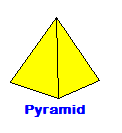CalcuNation

# Pyramid Volume Calculator

## Find the volume of a pyramid with this Pyramid Volume Calculator.

Base Area:

Height:

Fill in the area of the base, and the total pyramid height to calculate
volume.

### How do you find the volume of a pyramid?

To find the volume, use this pyramid volume formula:
Volume = 1/3 x Base Area x HeightExample: The volume of a pyramid with base area of 33 square inches
and height of 10 inches is:

Volume = 1/3 x 33 x 10

Calculated out this gives a volume of 110 Cubic Inches.

### \$100 Promotion

Win \$100 towards teaching supplies! We want to see your websites and blogs.Enter Here

### Calculator Popups

Scientific Calculator
Simple Calculator

### Calculator Ideas

We use your calculator ideas to create new and useful online calculators.
Submit Calculator Idea